# Population distribution VS Sampling distribution The population distribution

• Slides: 15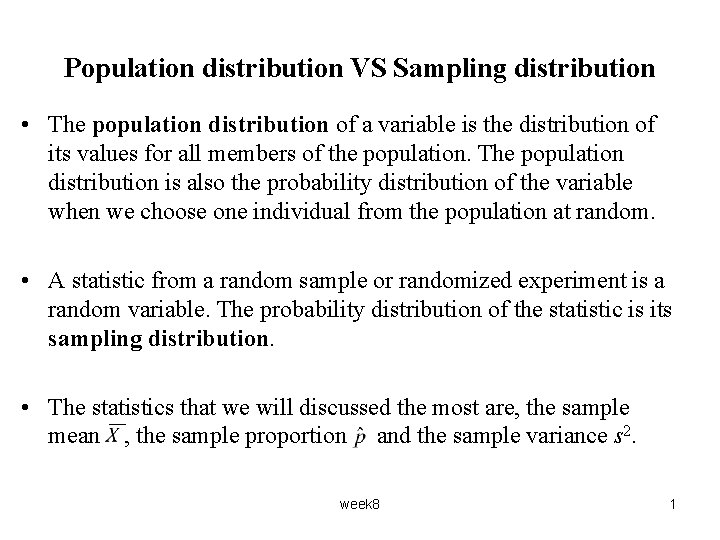Population distribution VS Sampling distribution • The population distribution of a variable is the distribution of its values for all members of the population. The population distribution is also the probability distribution of the variable when we choose one individual from the population at random. • A statistic from a random sample or randomized experiment is a random variable. The probability distribution of the statistic is its sampling distribution. • The statistics that we will discussed the most are, the sample mean , the sample proportion and the sample variance s 2. week 8 1The binomial distribution • The binomial setting: Ø There is a fixed number, n, of observations. Ø The n observations are independent. Ø Each observation falls into one of just two categories (“success” and “failures”). Ø The probability of a success (call it p) is the same for each observation. Ø The binomial r. v, X counts the number of successes in n trials. Notation: X ~ Bin(n, p). • Example: A biased coin (P(H) = p = 0. 6) ) is tossed 5 times. Let X be the number of H’s. Find P(X = 2). This X is a binomial r. v. week 8 2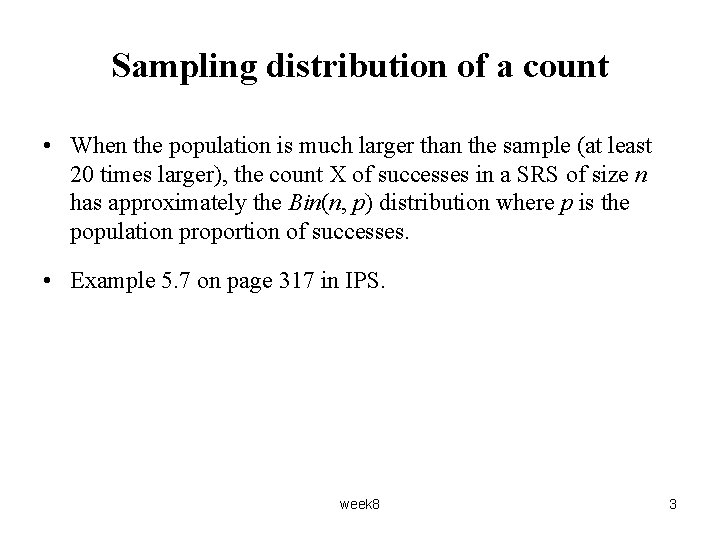Sampling distribution of a count • When the population is much larger than the sample (at least 20 times larger), the count X of successes in a SRS of size n has approximately the Bin(n, p) distribution where p is the population proportion of successes. • Example 5. 7 on page 317 in IPS. week 8 3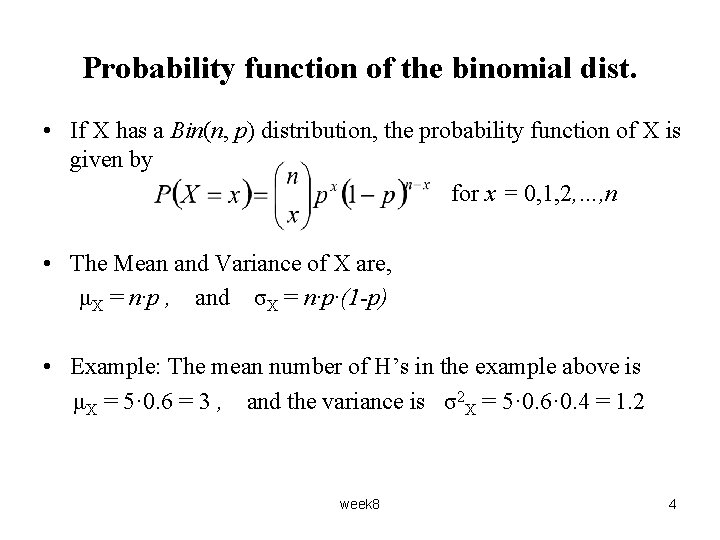Probability function of the binomial dist. • If X has a Bin(n, p) distribution, the probability function of X is given by for x = 0, 1, 2, …, n • The Mean and Variance of X are, μX = n·p , and σX = n·p·(1 -p) • Example: The mean number of H’s in the example above is μX = 5· 0. 6 = 3 , and the variance is σ2 X = 5· 0. 6· 0. 4 = 1. 2 week 8 4Example You are planning a sample survey of small businesses in your area. You will choose a SRS of businesses listed in the telephone book's Yellow Pages. Experience shows that only about half the businesses you contact will respond. (a) If you contact 150 businesses, it is reasonable to use the Bin(150, 0. 5) distribution for the number of businesses X who respond. Explain why. (b) What is the expected number (the mean) of businesses who will respond and what is its std dev. ? week 8 5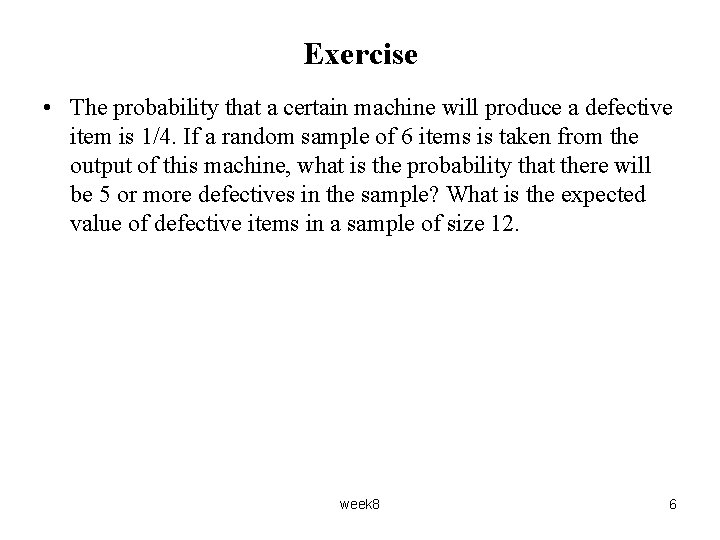Exercise • The probability that a certain machine will produce a defective item is 1/4. If a random sample of 6 items is taken from the output of this machine, what is the probability that there will be 5 or more defectives in the sample? What is the expected value of defective items in a sample of size 12. week 8 6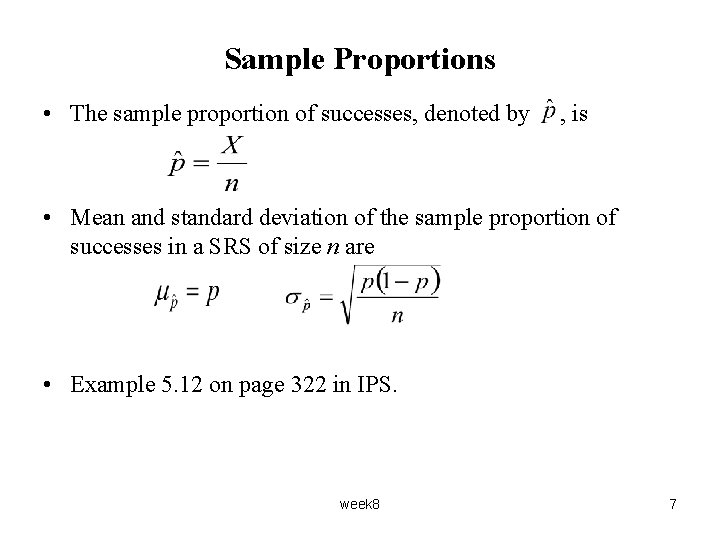Sample Proportions • The sample proportion of successes, denoted by , is • Mean and standard deviation of the sample proportion of successes in a SRS of size n are • Example 5. 12 on page 322 in IPS. week 8 7Question 1 Summer 2000, QIII b • Suppose that the ‘true’ odds are 6 to 4 that team A will win an upcoming Stanley Cup playoff series (so that probability of A winning is 0. 6). You place a bet in the amount of \$100 on team A, The payoff you will receive if team A wins is \$160. What is your expected net gain using the quoted odds above. • If the casino accepts 1000 bets just like yours, what is the expected income for the casino and the standard dev. of this income. week 8 8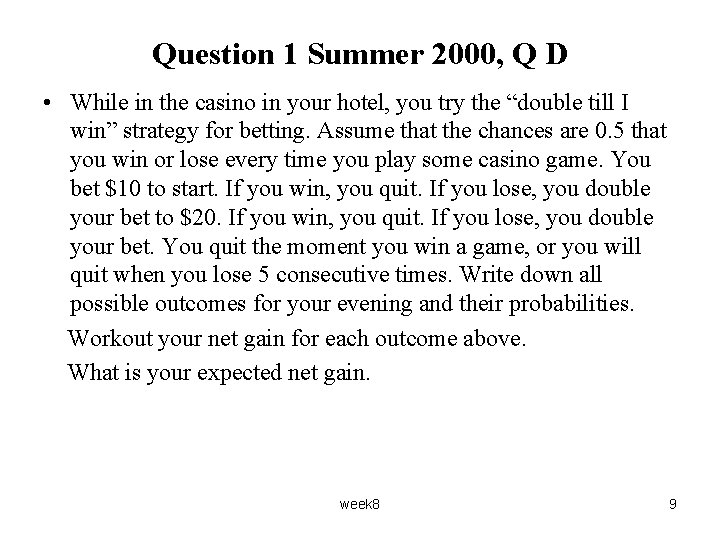Question 1 Summer 2000, Q D • While in the casino in your hotel, you try the “double till I win” strategy for betting. Assume that the chances are 0. 5 that you win or lose every time you play some casino game. You bet \$10 to start. If you win, you quit. If you lose, you double your bet to \$20. If you win, you quit. If you lose, you double your bet. You quit the moment you win a game, or you will quit when you lose 5 consecutive times. Write down all possible outcomes for your evening and their probabilities. Workout your net gain for each outcome above. What is your expected net gain. week 8 9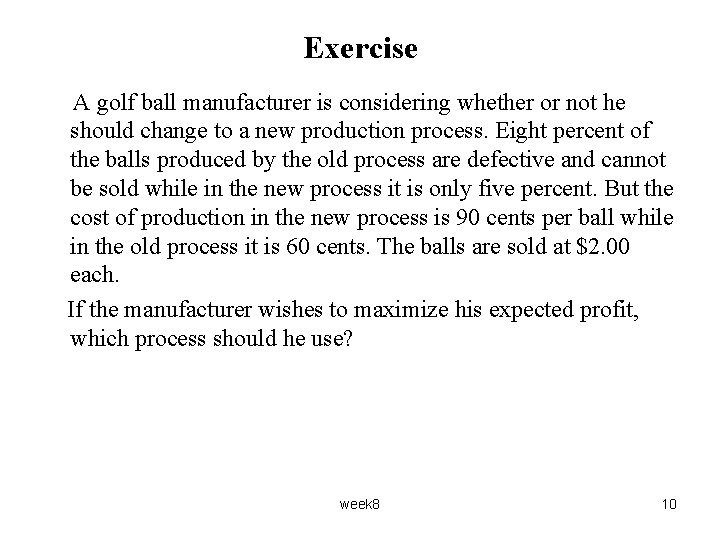Exercise A golf ball manufacturer is considering whether or not he should change to a new production process. Eight percent of the balls produced by the old process are defective and cannot be sold while in the new process it is only five percent. But the cost of production in the new process is 90 cents per ball while in the old process it is 60 cents. The balls are sold at \$2. 00 each. If the manufacturer wishes to maximize his expected profit, which process should he use? week 8 10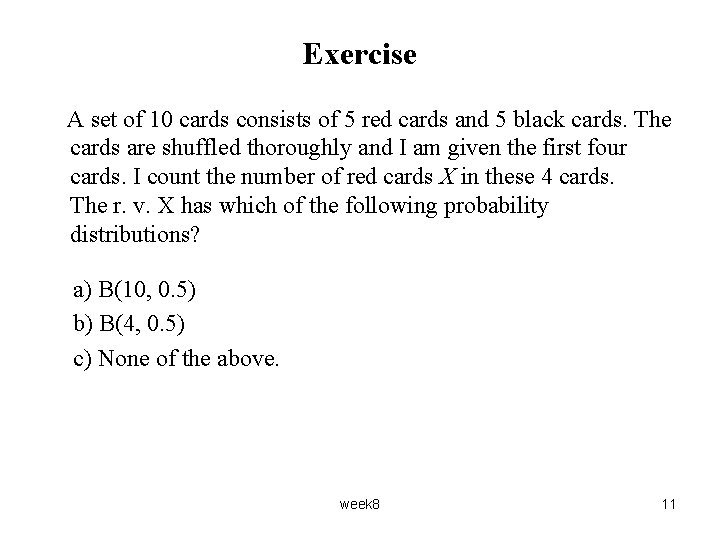Exercise A set of 10 cards consists of 5 red cards and 5 black cards. The cards are shuffled thoroughly and I am given the first four cards. I count the number of red cards X in these 4 cards. The r. v. X has which of the following probability distributions? a) B(10, 0. 5) b) B(4, 0. 5) c) None of the above. week 8 11Exercise • There are 20 multiple-choice questions on an exam, each having responses a, b, c, and d. Each question is worth 5 points. And only one response per question is correct. Suppose that a student guesses the answer to question and her guesses from question to question are independent. If the student needs at least 40 points to pass the test. What is the probability that the student will pass the test? • What is the expected (mean) score for this student. week 8 12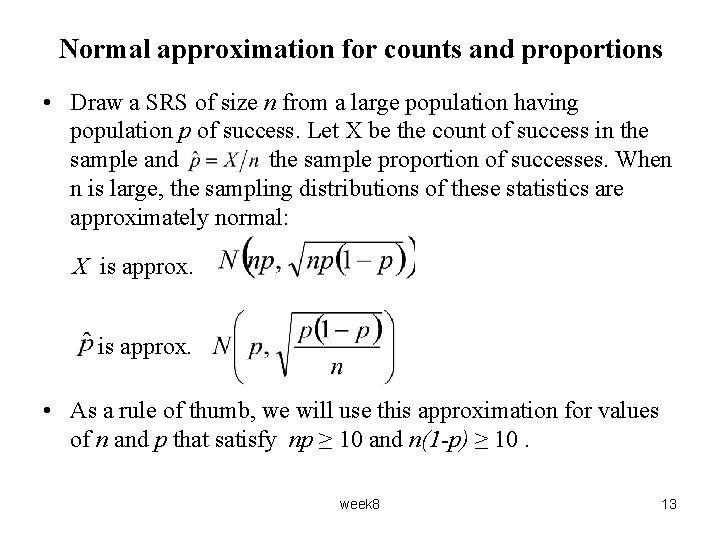Normal approximation for counts and proportions • Draw a SRS of size n from a large population having population p of success. Let X be the count of success in the sample and the sample proportion of successes. When n is large, the sampling distributions of these statistics are approximately normal: X is approx. • As a rule of thumb, we will use this approximation for values of n and p that satisfy np ≥ 10 and n(1 -p) ≥ 10. week 8 13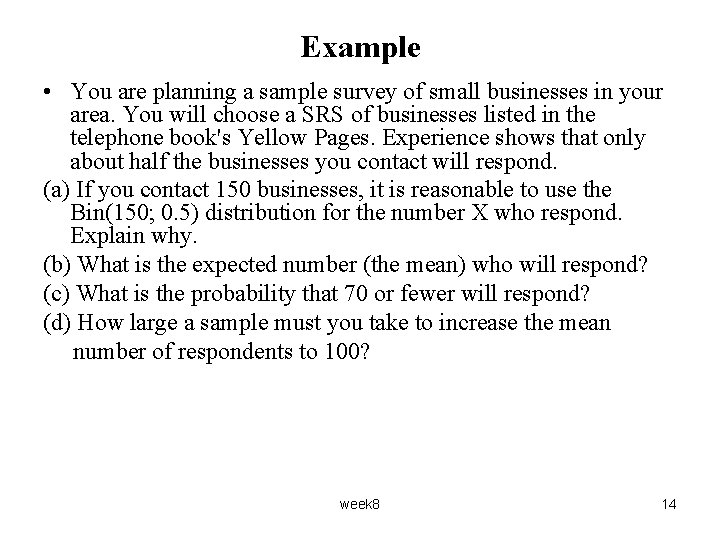Example • You are planning a sample survey of small businesses in your area. You will choose a SRS of businesses listed in the telephone book's Yellow Pages. Experience shows that only about half the businesses you contact will respond. (a) If you contact 150 businesses, it is reasonable to use the Bin(150; 0. 5) distribution for the number X who respond. Explain why. (b) What is the expected number (the mean) who will respond? (c) What is the probability that 70 or fewer will respond? (d) How large a sample must you take to increase the mean number of respondents to 100? week 8 14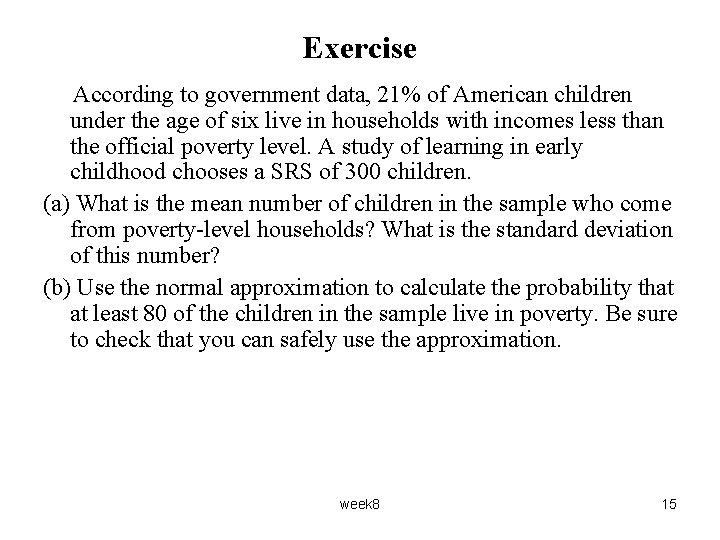Exercise According to government data, 21% of American children under the age of six live in households with incomes less than the official poverty level. A study of learning in early childhood chooses a SRS of 300 children. (a) What is the mean number of children in the sample who come from poverty-level households? What is the standard deviation of this number? (b) Use the normal approximation to calculate the probability that at least 80 of the children in the sample live in poverty. Be sure to check that you can safely use the approximation. week 8 15